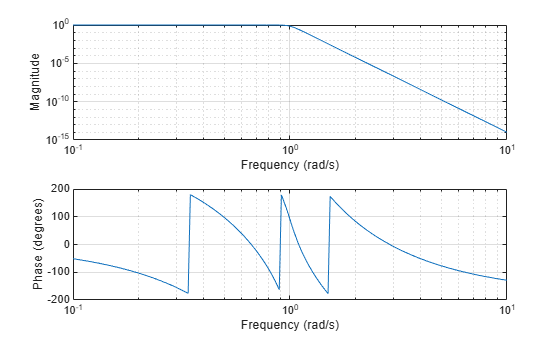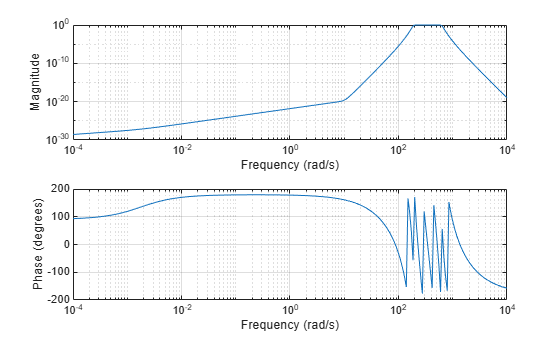# lp2bp

Transform lowpass analog filters to bandpass

## Syntax

``[bt,at] = lp2bp(b,a,Wo,Bw)``
``[At,Bt,Ct,Dt] = lp2bp(A,B,C,D,Wo,Bw)``

## Description

example

````[bt,at] = lp2bp(b,a,Wo,Bw)` transforms an analog lowpass filter prototype given by polynomial coefficients (specified by row vectors `b` and `a`) into a bandpass filter with center frequency `Wo` and bandwidth `Bw`. The input system must be an analog filter prototype.```
````[At,Bt,Ct,Dt] = lp2bp(A,B,C,D,Wo,Bw)` converts the continuous-time state-space lowpass filter prototype (specified by matrices `A`, `B`, `C`, and `D`) to a bandpass filter with center frequency `Wo` and bandwidth `Bw`. The input system must be an analog filter prototype.```

## Examples

collapse all

Design a 14th-order lowpass Butterworth analog filter prototype.

```n = 14; [z,p,k] = buttap(n);```

Convert the prototype to transfer function form. Display its magnitude and frequency responses.

```[b,a] = zp2tf(z,p,k); freqs(b,a)```Transform the prototype to a bandpass filter with a passband from 30 Hz to 100 Hz. Specify the center frequency and bandwidth in rad/s.

```fl = 30; fh = 100; Wo = 2*pi*sqrt(fl*fh); % center frequency Bw = 2*pi*(fh-fl); % bandwidth [bt,at] = lp2bp(b,a,Wo,Bw);```

Display the magnitude and frequency responses of the transformed filter.

`freqs(bt,at)`## Input Arguments

collapse all

Prototype numerator and denominator coefficients, specified as row vectors. `b` and `a` specify the coefficients of the numerator and denominator of the prototype in descending powers of s:

`$\frac{B\left(s\right)}{A\left(s\right)}=\frac{b\left(1\right){s}^{n}+\cdots +b\left(n\right)s+b\left(n+1\right)}{a\left(1\right){s}^{m}+\cdots +a\left(m\right)s+a\left(m+1\right)}$`

Data Types: `single` | `double`

Prototype state-space representation, specified as matrices. The state-space matrices relate the state vector x, the input u, and the output y through

`$\begin{array}{l}\stackrel{˙}{x}=Ax+Bu\\ y=Cx+Du\end{array}$`

Data Types: `single` | `double`

Center frequency, specified as a scalar. For a filter with lower band edge `w1` and upper band edge `w2`, use `Wo` = sqrt(`w1`*`w2`). Express `Wo` in units of rad/s.

Data Types: `single` | `double`

Bandwidth, specified as a scalar. For a filter with lower band edge `w1` and upper band edge `w2`, use `Bw` = `w2``w1`. Express `Bw` in units of rad/s.

Data Types: `single` | `double`

## Output Arguments

collapse all

Transformed numerator and denominator coefficients, returned as row vectors.

Transformed state-space representation, returned as matrices.

## Algorithms

`lp2bp` transforms analog lowpass filter prototypes with a cutoff angular frequency of 1 rad/s into bandpass filters with the desired bandwidth and center frequency. The transformation is one step in the digital filter design process for the `butter`, `cheby1`, `cheby2`, and `ellip` functions.

`lp2bp` is a highly accurate state-space formulation of the classic analog filter frequency transformation. Consider the state-space system

`$\begin{array}{l}\stackrel{˙}{x}=Ax+Bu\\ y=Cx+Du\end{array}$`

where u is the input, x is the state vector, and y is the output. The Laplace transform of the first equation (assuming zero initial conditions) is

`$sX\left(s\right)=AX\left(s\right)+BU\left(s\right)$`

Now if a bandpass filter has center frequency ω0 and bandwidth Bw, the standard s-domain transformation is

`$s=Q\left({p}^{2}+1\right)/p$`

where Q = ω0/Bw and p = s0. Substituting this for s in the Laplace transformed state-space equation and considering the operator p as d/dt results in

`$Q\stackrel{¨}{x}+Qx=\stackrel{˙}{A}x+B\stackrel{˙}{u}$`

or

`$Q\stackrel{¨}{x}-\stackrel{˙}{A}x-B\stackrel{˙}{u}=-Qx$`

Now define

`$Q\stackrel{˙}{\omega }=-Qx$`

`$Q\stackrel{˙}{x}=Ax+Q\omega +Bu$`

The last two equations give equations of state. Write them in standard form and multiply the differential equations by ω0 to recover the time or frequency scaling represented by p and find state matrices for the bandpass filter:

`$Q=\frac{{\omega }_{0}}{{B}_{w}}$`
`$At={\omega }_{0}\left[\frac{A\text{\hspace{0.17em}}}{Q}\text{\hspace{0.17em}}\text{\hspace{0.17em}}\mathrm{eye}\left(ma,m\right);-\mathrm{eye}\left(ma,m\right)\text{\hspace{0.17em}}\text{\hspace{0.17em}}\mathrm{zeros}\left(ma,m\right)\right]$`
`$Bt={\omega }_{0}\left[\frac{B}{Q};\mathrm{zeros}\left(ma,n\right)\right]$`
`$Ct=\left[C\text{\hspace{0.17em}}\mathrm{zeros}\left(mc,ma\right)\right]$`
`$Dt=D$`

where $\left[ma,m\right]=\mathrm{size}\left(A\right)$.

`lp2bp` can perform the transformation on two different linear system representations: transfer function form and state-space form. If the input to `lp2bp` is in transfer function form, the function transforms it into state-space form before applying this algorithm.

## Version History

Introduced before R2006a